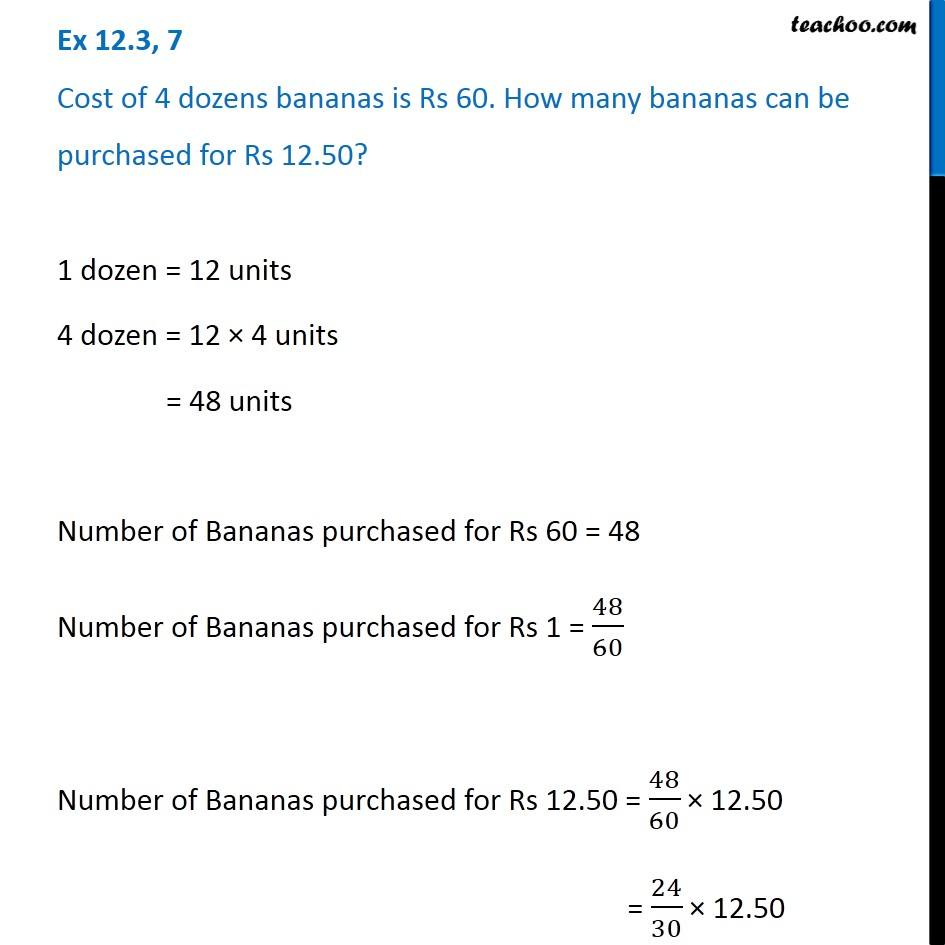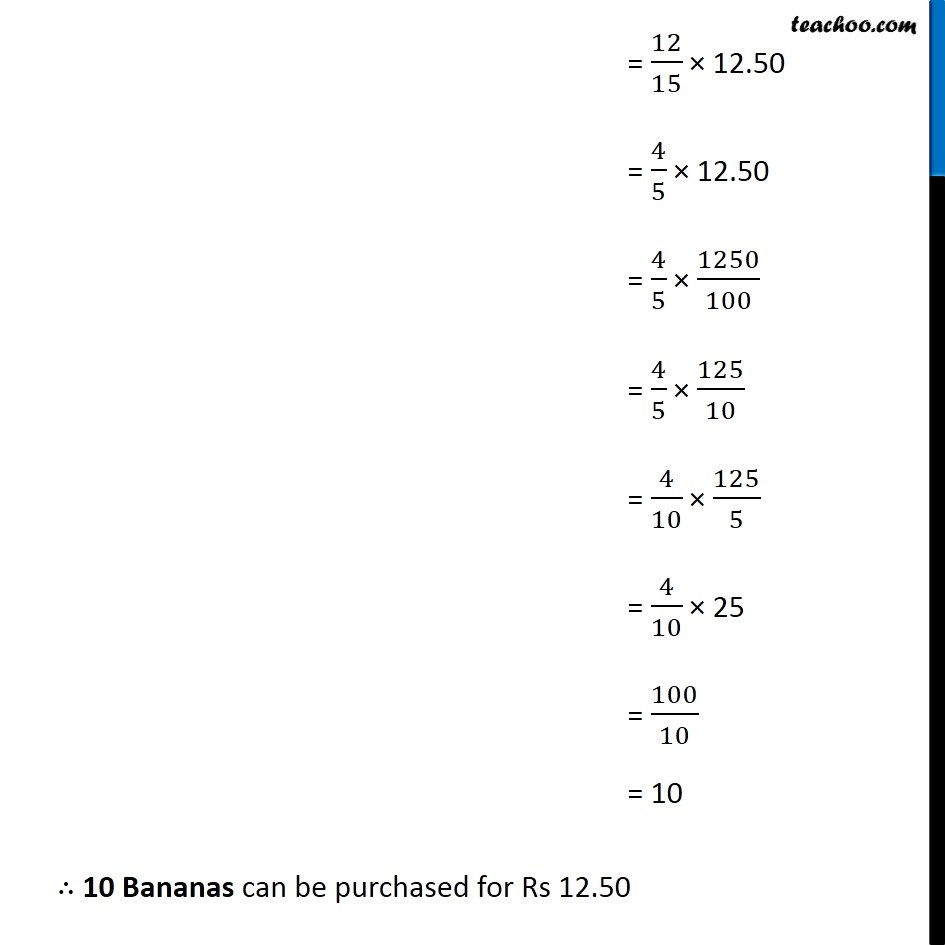Ex 12.3

Chapter 12 Class 6 Ratio and Proportion
Serial order wiseLearn in your speed, with individual attention - Teachoo Maths 1-on-1 Class

### Transcript

Ex 12.3, 7 Cost of 4 dozens bananas is Rs 60. How many bananas can be purchased for Rs 12.50? 1 dozen = 12 units 4 dozen = 12 × 4 units = 48 units Number of Bananas purchased for Rs 60 = 48 Number of Bananas purchased for Rs 1 = 48/60 Number of Bananas purchased for Rs 12.50 = 48/60 × 12.50 = 24/30 × 12.50 = 12/15 × 12.50 = 4/5 × 12.50 = 4/5 × 1250/100 = 4/5 × 125/10 = 4/10 × 125/5 = 4/10 × 25 = 100/10 = 10 ∴ 10 Bananas can be purchased for Rs 12.50